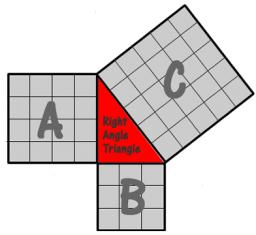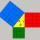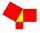# Is right?

Determine whether the triangle with legs (catheti) 19.5 cm and 26 cm and length of the hypotenuse 32.5 cm is rectangular?

x =  1

### Step-by-step explanation:Did you find an error or inaccuracy? Feel free to write us. Thank you!Tips to related online calculators
Pythagorean theorem is the base for the right triangle calculator.

#### You need to know the following knowledge to solve this word math problem:

We encourage you to watch this tutorial video on this math problem:

## Related math problems and questions:

• HypotenuseCalculate the length of the hypotenuse of a right triangle with a catheti 71 cm and 49 cm long.
• Median in right triangleIn the rectangular triangle ABC has known the length of the legs a = 15cm and b = 36cm. Calculate the length of the median to side c (to hypotenuse).
• IS trapezoidCalculate the length of diagonal u and height v of isosceles trapezoid ABCD, whose bases have lengths a = |AB| = 37 cm, c = |CD| = 29 cm and legs b = d = |BC| = |AD| = 28 cm.
• OPTWhat is the perimeter of a right triangle with the legs 14 cm and 21 cm long?
• RTriangle 17The hypotenuse of a right triangle is 17 cm. If you decrease both two legs by 3 cm you will reduce the hypotenuse by 4 cm. Determine the length of this legs.
• Right triangleLegs of the right triangle are in the ratio a:b = 2:8. The hypotenuse has a length of 87 cm. Calculate the perimeter and area of the triangle.
• DiagonalsA diagonal of a rhombus is 20 cm long. If it's one side is 26 cm, find the length of the other diagonal.
• Rectangular triangle PQRIn the rectangular triangle PQR, the PQ leg is divided by the X point into two segments of which longer is 25cm long. The second leg PR has a length 16 cm. The length of the RX is 20 cm. Calculate the length p of side RQ. The result is round to 2 decimal
• RectangleThe rectangle is 21 cm long and 38 cm wide. Determine the radius of the circle circumscribing rectangle.
• CalculateCalculate the height to the base of the isosceles triangle ABC if the length of the base is c = 24cm and the arms have a length b = 13cm.
• CathetiThe hypotenuse of a right triangle is 41 and the sum of legs is 49. Calculate the length of its legs.
• Triangle KLMIn the rectangular triangle KLM, where is hypotenuse m (sketch it!). Find the length of the leg k and the height of triangle h if the hypotenuse's segments are known MK = 5cm and ml = 15 cm.
• Right angled triangleThe hypotenuse of a right triangle is 17 cm long. When we decrease the length of legs by 3 cm, then decrease its hypotenuse by 4 cm. Find the size of its legs.
• Area of RTIn the right triangle has orthogonal projections of legs to the hypotenuse lengths 15 cm and 9 cm. Determine the area of ​​this triangle.
• Sum of squaresThe sum of squares above the sides of the rectangular triangle is 900 cm2. Calculate content of square over the triangle's hypotenuse.
• Right triangle - legCalculate to the nearest tenth cm length of leg in right-angled triangle with hypotenuse length 9 cm and 7 cm long leg.
• R TrapeziumRectangular trapezium has bases 21 and 10 and area 92 cm2. What is its perimeter?